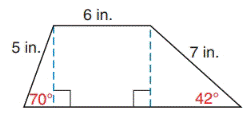Chapter 11.4, Problem 16EElementary Geometry For College St...

7th Edition
Alexander + 2 others
ISBN: 9781337614085

Solutions

Chapter
SectionElementary Geometry For College St...

7th Edition
Alexander + 2 others
ISBN: 9781337614085
Textbook Problem

In Exercises 15 and 16, find the area of the given figure. Give the answer to the nearest tenth of a square unit.Trapezoid

To determine

To find:

The area of the given figure

Explanation

Approach:

Finding the area of the figure is followed by identifying the figure and find area with the suitable geometry formulae.

Calculation:

Given,

The area of the given figure (A)= The area of the triangle (MRQ)(A1)+ The area of the square RSPQ(A2)+ The area of the triangle SNP(A3)

The area of the triangle (MRQ)(A1):

For ΔRMQ

α+β+γ=180

Here α=70 and γ=90

70+β+90=180

160+β=180

Add 160 on both sides,

160+β160=180160

β=20

Here,

β=20

a=5 in.,

c=6 in.

Area of the triangle (A1)=12acsinβ

12(5)(6)sin20

=(5)(3)sin20

=(15)sin20

=(15)(0.34)

A1=5.1 in2.

The area of the square (RSPQ)(A2):

Side (s)=6 in.

Still sussing out bartleby?

Check out a sample textbook solution.

See a sample solution

The Solution to Your Study Problems

Bartleby provides explanations to thousands of textbook problems written by our experts, many with advanced degrees!

Get Started

Find more solutions based on key concepts